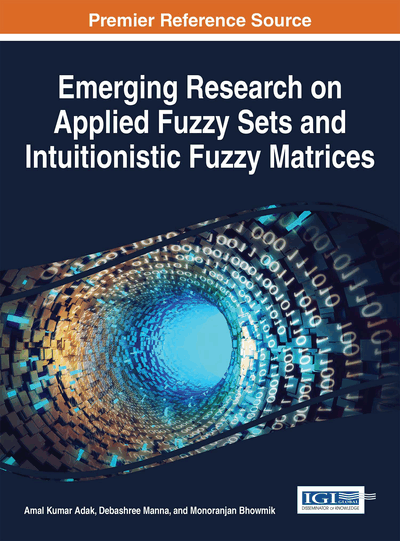# Semiring of Generalized Interval-Valued Intuitionistic Fuzzy Matrices

Debashree Manna (Damda Jr. High School, India)
DOI: 10.4018/978-1-5225-0914-1.ch006
Available
\$37.50
No Current Special Offers

## Abstract

In this paper, the concept of semiring of generalized interval-valued intuitionistic fuzzy matrices are introduced and have shown that the set of GIVIFMs forms a distributive lattice. Also, prove that the GIVIFMs form an generalized interval valued intuitionistic fuzzy algebra and vector space over [0, 1]. Some properties of GIVIFMs are studied using the definition of comparability of GIVIFMs.
Chapter Preview
Top

## Preliminaries

Here some preliminaries, definitions of IVIFMs and GIVIFMs are recalled and presented some operations on GIVIFMs.

### Definition 1

A semiring is an algebraic structure (R,+,.) such that (R,+) is an abelian monoid (identity 0), (R,.) is a monoid (identity 1). distributes over + from either side, r0=0r=0 for all rR and 0≠1.

### Definition 2

A fuzzy matrix (FM) of order m×n is defined aswhereis the membership value of the ij-element in A. Letdenote the set of all fuzzy matrices of order m×n. If m=n, in short, we write Fn, the set of all square matrices of order n.

## Complete Chapter List

Search this Book:
Reset